Author: Oscar Cronquist Article last updated on June 09, 2022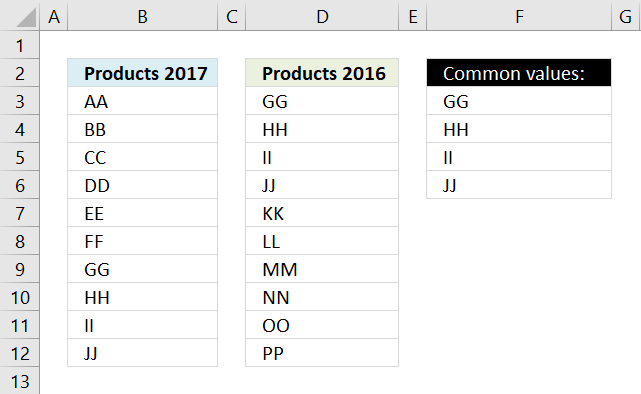This article demonstrates ways to extract shared values in different cell ranges, two and three cell ranges. The Excel 365 formulas are smaller and easier to understand.

## 1. Extract shared values between two columnsQuestion: How can I compare two columns to find values that exist in both cell ranges?

The picture above shows two lists, one in column B and one in column D. The array formula in cell F3 extracts values that both lists have.

Array formula in cell F3:

=INDEX(\$B\$3:\$B\$12, SMALL(IF(COUNTIF(\$D\$3:\$D\$12, \$B\$3:\$B\$12), MATCH(ROW(\$B\$3:\$B\$12),ROW(\$B\$3:\$B\$12)), ""), ROWS(\$A\$1:A1)))

In this case GG, HH, II, and JJ are in both lists, see the picture below.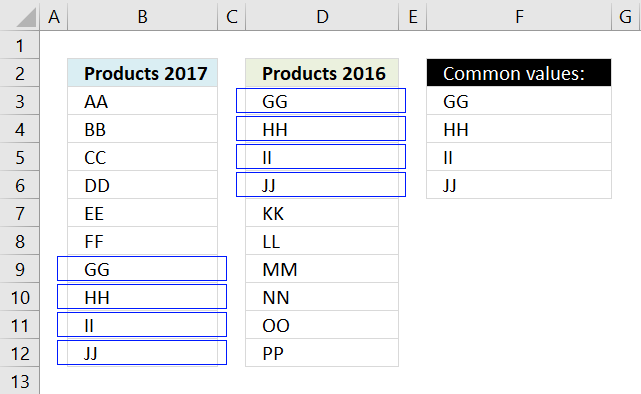The formula above can only compare two columns, however, the lists don't have to be the same size.

If you need to compare two different multicolumn cell ranges, read the following article:

Recommended articles

Filter common values between two ranges
The image above shows an array formula in cell B12 that extracts values shared by cell range B2:D4 (One) and […]

### 1.1 How to create an array formula

1. Select cell F3
2. Press with left mouse button on in formula bar3. Copy and paste the array formula above to formula bar
4. Press and hold Ctrl + Shift simulateously
5. Press Enter
6. Release all keys

You can check using the formula bar that you did above steps right, excel tells you if a cell contains an array formula by surrounding the formula with a beginning and ending curly brackets, like this: {=array_formula}.

Don't enter these characters yourself they show up automatically if you did above steps correctly.

Recommended articles

A beginners guide to Excel array formulas
Array formulas allows you to do advanced calculations not possible with regular formulas.

### 1.2 How to copy array formula

Copy cell F3 and paste it to cells below as far as needed.

### 1.3 Explaining array formula in cell C2

You can easily examine a formula (or array formula) that you don't understand, select the cell containing the formula. Go to tab "Formulas", press with left mouse button on "Evaluate Formula".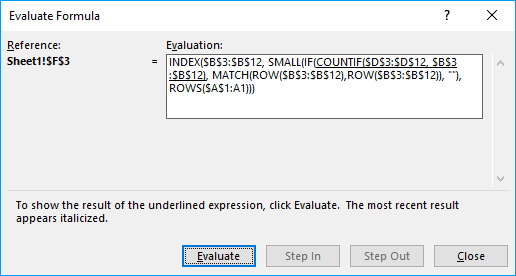The "Evaluate" button above lets you see the next "calculation" step.

#### Step 1 - Compare cell range 1 with cell range 2

The COUNTIF function lets you compare values if you enter it as an array formula and use multiple values as criteria. COUNTIF(range, criteria)

COUNTIF(\$D\$3:\$D\$12, \$B\$3:\$B\$12)

becomes

COUNTIF({"GG"; "HH"; "II"; "JJ"; "KK"; "LL"; "MM"; "NN"; "OO"; "PP"}, {"AA"; "BB"; "CC"; "DD"; "EE"; "FF"; "GG"; "HH"; "II"; "JJ"})

and returns

{0; 0; 0; 0; 0; 0; 1; 1; 1; 1}

The array is shown in column H below.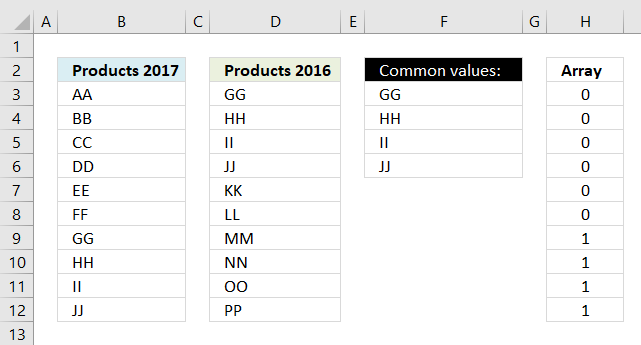This tells us that AA exists 0 (zero) times in cell range D3:D12,
BB - 0, CC - 0, DD - 0, EE-0, FF - 0

but GG is found once in cell range D3:D12 and so are HH, II, JJ.

Recommended articles

How to use the COUNTIF function
Counts the number of cells that meet a specific condition.

#### Step 2 - Check if value exists, if so return corresponding position in array

IF({0; 0; 0; 0; 0; 0; 1; 1; 1; 1}, MATCH(ROW(\$B\$3:\$B\$12),ROW(\$B\$3:\$B\$12)), "")

becomes

IF({0; 0; 0; 0; 0; 0; 1; 1; 1; 1}, {1; 2; 3; 4; 5; 6; 7; 8; 9; 10}, "")

and returns

{""; ""; ""; ""; ""; ""; 7; 8; 9; 10}

The array is shown in column H below.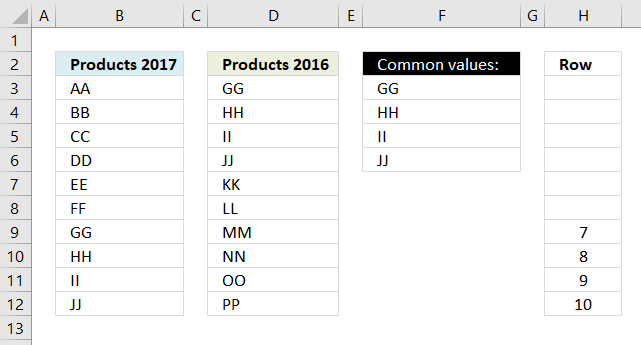Recommended articles

How to use the IF function
Checks if a logical expression is met. Returns a specific value if TRUE and another specific value if FALSE.

#### Step 3 - Extract k-th smallest value

Until now we have been working with an array of values but excel allows us to only display one value per cell (That is not entirely true, as of Excel 2016 you can display all values in an array in one cell)

To extract a specific number from an array I use the SMALL function.

SMALL(IF(COUNTIF(\$D\$3:\$D\$12, \$B\$3:\$B\$12), MATCH(ROW(\$B\$3:\$B\$12),ROW(\$B\$3:\$B\$12)), ""), ROWS(\$A\$1:A1))

becomes

SMALL({""; ""; ""; ""; ""; ""; 7; 8; 9; 10}, ROWS(\$A\$1:A1))

becomes

SMALL({""; ""; ""; ""; ""; ""; 7; 8; 9; 10}, 1)

and returns number 7, SMALL function ignores blanks and letters.

Recommended articles

How to use the SMALL function
The SMALL function lets you extract a number in a cell range based on how small it is compared to the other numbers in the group.

#### Step 4 - Return corresponding value

INDEX(\$B\$3:\$B\$12, SMALL(IF(COUNTIF(\$D\$3:\$D\$12, \$B\$3:\$B\$12), MATCH(ROW(\$B\$3:\$B\$12),ROW(\$B\$3:\$B\$12)), ""), ROWS(\$A\$1:A1)))

becomes

INDEX(\$B\$3:\$B\$12, 7)

becomes

INDEX({"AA"; "BB"; "CC"; "DD"; "EE"; "FF"; "GG"; "HH"; "II"; "JJ"}, 7)

and returns GG in cell F3.When you copy cell F3 and paste it to cell F4 the relative cell references changes. ROWS(\$A\$1:A1) becomes ROWS(\$A\$1:A2) and returns 2 in cell F4.

The second smallest value is then extracted from the array which is 8. The value in cell range B3:B12 in row 8 is HH. HH is returned the value returned to F4.

Recommended articles

How to use the INDEX function
Gets a value in a specific cell range based on a row and column number.

### Get excel sample file for this tutorial

common-values1.xlsx
(Excel 2007 Workbook *.xlsx and later versions)

## 2. Extract shared values between two columns - Excel 365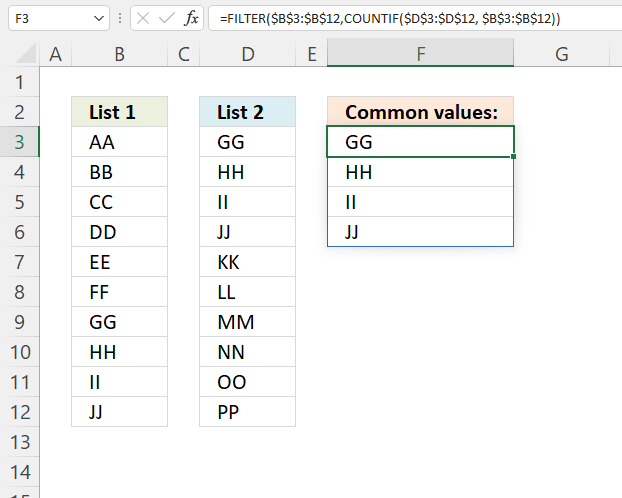This Excel 365 dynamic array formula extracts values from cell range B3:B12 only if they also exist in cell range D3:D12.

Formula in cell F3:

=FILTER(\$B\$3:\$B\$12,COUNTIF(\$D\$3:\$D\$12, \$B\$3:\$B\$12))

### 2.1 Explaining formula

#### Step 1 - Find values in common

The COUNTIF function lets you compare values if you enter it as an array formula and use multiple values as criteria.

COUNTIF(range, criteria)

COUNTIF(\$D\$3:\$D\$12, \$B\$3:\$B\$12)

becomes

COUNTIF({"GG"; "HH"; "II"; "JJ"; "KK"; "LL"; "MM"; "NN"; "OO"; "PP"}, {"AA"; "BB"; "CC"; "DD"; "EE"; "FF"; "GG"; "HH"; "II"; "JJ"})

and returns

{0; 0; 0; 0; 0; 0; 1; 1; 1; 1}

COUNTIF(\$D\$3:\$D\$12, \$B\$3:\$B\$12)

#### Step 2 - Extract values

The FILTER function extracts values/rows based on a condition or criteria.

FILTER(arrayinclude, [if_empty])

FILTER(\$B\$3:\$B\$12,COUNTIF(\$D\$3:\$D\$12, \$B\$3:\$B\$12))

becomes

FILTER(\$B\$3:\$B\$12, {0; 0; 0; 0; 0; 0; 1; 1; 1; 1})

and returns

{"GG"; "HH"; "II"; "JJ"}

## 3. Extract shared values between two columns - case sensitive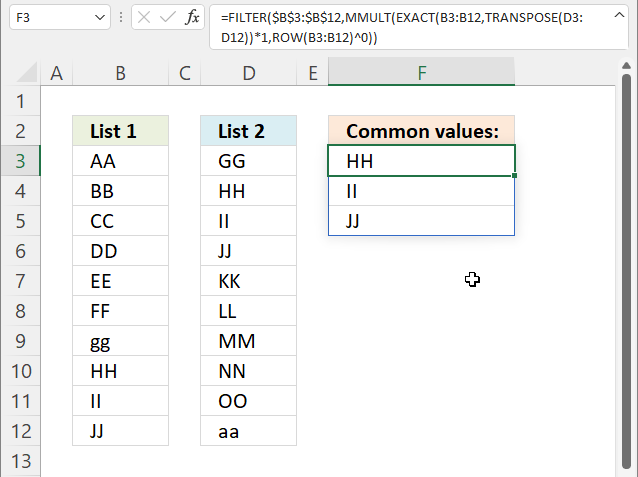This formula shown in the image above extracts values in the first cell range if they also exist in the second cell range, upper and lower letters are also evaluated.

Excel 365 dynamic array formula in cell F3:

=FILTER(\$B\$3:\$B\$12,MMULT(EXACT(B3:B12,TRANSPOSE(D3:D12))*1,ROW(B3:B12)^0))

### 3.1 Explaining formula

#### Step 1 - Rearrange values from vertical to horizontal

The TRANSPOSE function converts a vertical range to a horizontal range, or vice versa.

TRANSPOSE(array)

TRANSPOSE(D3:D12)

becomes

TRANSPOSE({"GG"; "HH"; "II"; "JJ"; "KK"; "LL"; "MM"; "NN"; "OO"; "aa"})

and returns

{"GG", "HH", "II", "JJ", "KK", "LL", "MM", "NN", "OO", "aa"}.

#### Step 2 - Compare values based on upper and lower letters

The EXACT function performs a case sensitive comparison between values.

EXACT(value1, value2)

EXACT(B3:B12,TRANSPOSE(D3:D12))

becomes

EXACT(B3:B12,{"GG", "HH", "II", "JJ", "KK", "LL", "MM", "NN", "OO", "aa"})

becomes

EXACT({"AA"; "BB"; "CC"; "DD"; "EE"; "FF"; "gg"; "HH"; "II"; "JJ"}, {"GG", "HH", "II", "JJ", "KK", "LL", "MM", "NN", "OO", "aa"})

and returns an array shown in the image below. I have added the corresponding values from both cell ranges and highlighted values that exist in both cell ranges.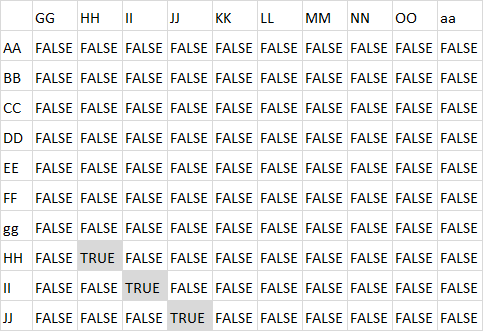#### Step 3 - Convert boolean values

The asterisk lets you multiply numbers in an Excel formula, it also lets you convert boolean values to their numerical equivalents.

TRUE -> 1
FALSE -> 0 (zero)

EXACT(B3:B12,TRANSPOSE(D3:D12))*1

becomes

{FALSE,FALSE,FALSE, ... ,FALSE}*1

and returns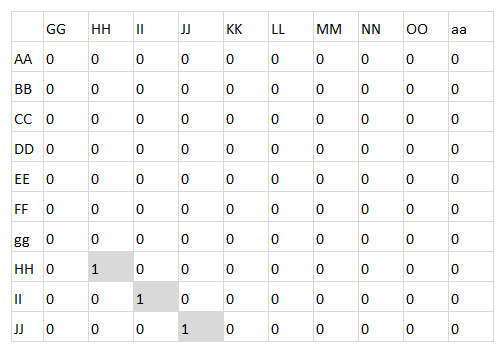#### Step 4 - Create an array containing 1's

The ROW function returns the corresponding row number in a cell reference or multiple row numbers if a cell range reference is used.

ROW(reference)

ROW(B3:B12)^0

becomes

{3; 4; 5; 6; 7; 8; 9; 10; 11; 12}^0

and returns

{1; 1; 1; 1; 1; 1; 1; 1; 1; 1}.

#### Step 5 - Sum numbers row-wise

The MMULT function calculates the matrix product of two arrays, an array as the same number of rows as array1 and columns as array2.

MMULT(array1, array2)

MMULT(EXACT(B3:B12,TRANSPOSE(D3:D12))*1,ROW(B3:B12)^0)

becomes

MMULT({0,0,0, ... ,0},{1; 1; 1; 1; 1; 1; 1; 1; 1; 1})

and returns

{0; 0; 0; 0; 0; 0; 0; 1; 1; 1}.

#### Step 6 - Filter values based on array

The FILTER function extracts values/rows based on a condition or criteria.

FILTER(arrayinclude, [if_empty])

FILTER(\$B\$3:\$B\$12,MMULT(EXACT(B3:B12,TRANSPOSE(D3:D12))*1,ROW(B3:B12)^0))

becomes

FILTER(\$B\$3:\$B\$12, {0; 0; 0; 0; 0; 0; 0; 1; 1; 1})

and returns

{"HH"; "II"; "JJ"}.

## 4. Filter common values from three separate columns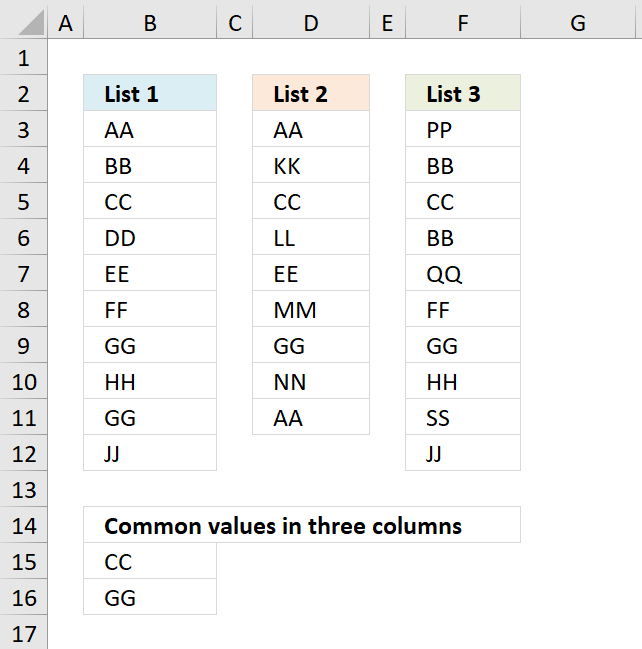The image above demonstrates a formula in cell B15 that extracts values if they exist in all three cell ranges B3:B12, D3:D12, and F3:F12.

Array formula in B15:

=INDEX(\$B\$3:\$B\$12, MATCH(0, COUNTIF(\$B\$14:B14, \$B\$3:\$B\$12)+IF(((COUNTIF(\$D\$3:\$D\$11, \$B\$3:\$B\$12)>0)+(COUNTIF(\$F\$3:\$F\$12, \$B\$3:\$B\$12)>0))=2, 0, 1), 0))

Copy cell B15 and paste it to cells below as far as necessary.

To enter an array formula, type the formula in a cell then press and hold CTRL + SHIFT simultaneously, now press Enter once. Release all keys.

The formula bar now shows the formula with a beginning and ending curly bracket telling you that you entered the formula successfully. Don't enter the curly brackets yourself.

### Explaining formula in cell B15

#### Step 1 - Prevent duplicates in the list

The COUNTIF function counts values based on a condition or criteria.  The first argument \$B\$14:B14 expands as the cell is copied to cells below. This makes the formula aware of displayed values above the current cell.

COUNTIF(\$B\$14:B14, \$B\$3:\$B\$12)

becomes

COUNTIF("Common values in three columns", {"AA"; "BB"; "CC"; "DD"; "EE"; "FF"; "GG"; "HH"; "GG"; "JJ"})

and returns

{0;0;0;0;0;0;0;0;0;0}

#### Step 2 - Find position of value in array

The MATCH function returns a number representing the position of a value in a list.

MATCH(0,COUNTIF(\$B\$14:B14,\$B\$3:\$B\$12)+(((COUNTIF(\$D\$3:\$D\$11,\$B\$3:\$B\$12)>0)+(COUNTIF(\$F\$3:\$F\$12,\$B\$3:\$B\$12)>0))<>2),0)

becomes

MATCH(0,C{0;0;0;0;0;0;0;0;0;0}+((({TRUE;FALSE;TRUE;FALSE;TRUE;FALSE;TRUE;FALSE;TRUE;FALSE})+({FALSE;TRUE;TRUE;FALSE;FALSE;TRUE;TRUE;TRUE;TRUE;TRUE}))<>2),0)

becomes

MATCH(0,{1;1;0;1;1;1;0;1;0;1},0)

and returns 3.

#### Step 3 - Return value

The INDEX function returns a value based on a row and column number.

INDEX(\$B\$3:\$B\$12, MATCH(0, COUNTIF(\$B\$14:B14, \$B\$3:\$B\$12)+IF(((COUNTIF(\$D\$3:\$D\$11, \$B\$3:\$B\$12)>0)+(COUNTIF(\$F\$3:\$F\$12, \$B\$3:\$B\$12)>0))=2, 0, 1), 0))

becomes

INDEX(\$B\$3:\$B\$12, 3)

and returns "CC" in cell B15.

### Get Excel *.xlsx file

Common values in three columns.xlsx